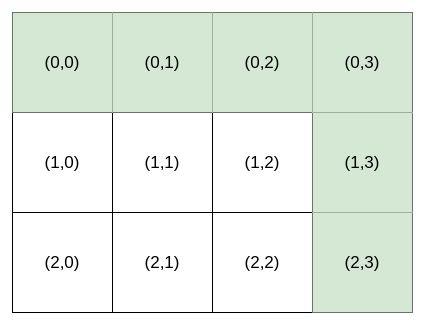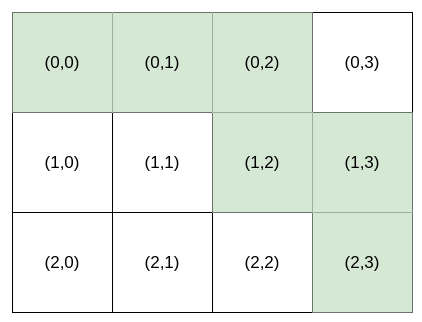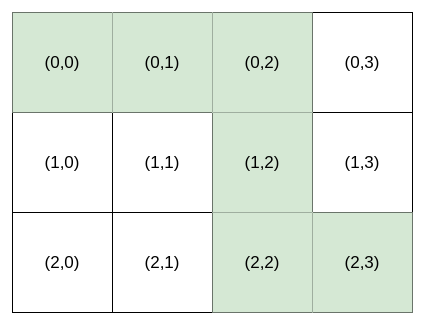# 第 K 条最小指令

## 1643. 第 K 条最小指令 (Hard)

Bob 站在单元格 `(0, 0)` ，想要前往目的地 `destination``(row, column)` 。他只能向 或向 走。你可以为 Bob 提供导航 指令 来帮助他到达目的地 `destination`

• `'H'` ，意味着水平向右移动
• `'V'` ，意味着竖直向下移动```输入：destination = [2,3], k = 1

["HHHVV", "HHVHV", "HHVVH", "HVHHV", "HVHVH", "HVVHH", "VHHHV", "VHHVH", "VHVHH", "VVHHH"].
``````输入：destination = [2,3], k = 2

``````输入：destination = [2,3], k = 3

```

• `destination.length == 2`
• `1 <= row, column <= 15`
• `1 <= k <= nCr(row + column, row)`，其中 `nCr(a, b)` 表示组合数，即从 `a` 个物品中选 `b` 个物品的不同方案数。

[动态规划]